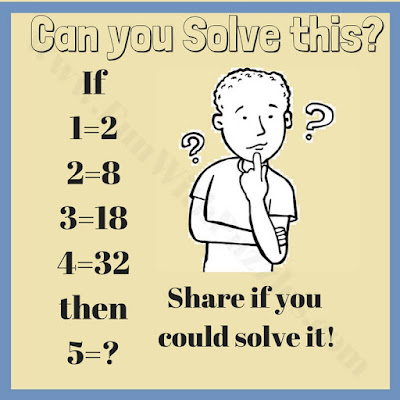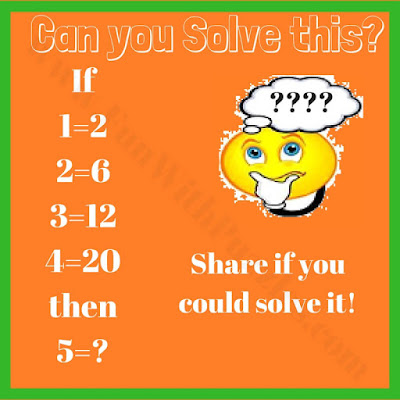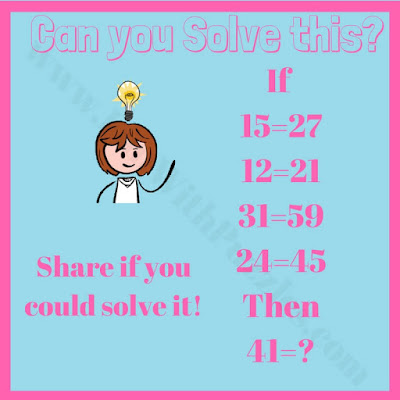Mathematics as a subject is very much if you know the fundamentals of mathematics very well. I found many students loving it and there are many students who do not like Mathematics as a subject at all. The difference comes in understanding the fundamentals of this subject. If Mathematics can be thought in an interesting way then it's always fun to solve mathematical problems. It becomes great fun to solve mathematically logical problems. Here are some of the math logic problems for high school students. Hopefully, it will be fun learning for students.

## How to Solve Logic Maths Puzzles

There are five mathematical logical problems. In these puzzles, few IF statements are given and one has to solve this IF pattern and after solving this one has to solve the THEN statement. Let us see how many of these puzzles you can solve quickly?1. Math Logic Number Brain Teaser for Teens2. Logical Math Mind Twister Riddle for Students3. Math Logic Number Equation Puzzle Question5. Math Logical Puzzle for Teens in High School

## Answers to Math Logic Brain Teasers

Unknown said...

Anyone solved #3? I cannot figure it out and wonder if there is a mistake.... :)

Rajesh Kumar said...

Answer for #3 is 30. Logic in this puzzle is A*(A+1)=B

Rajesh Kumar said...

Bala said...

50

Bala said...

2-30
3-40

Unknown said...

Unknown said...

ans
for 1 is 50
for 2 is 129
for 3 is 30
for 4 is 40
for 5 is 79

Unknown said...

I disagree. For 2 should be 1 because if 1=5, then 5=1.

Unknown said...

1=4
5=35

naomi said...

I think that it is 50 by the way I am eleven

Fun With Puzzles said...

@Naomi,
Nice to see you solving puzzles and brainteasers at the small age of eleven. Hope you enjoyed the puzzles on this website. Looking forward to more comments and feedback from you.

Anonymous said...

I do these even though I am in 5th grade

Anonymous said...

I am in Elemantery 5th grade and solve these without spending more then 30 sec. Maybe trey renaming the title.

Viet Giap (Tony) said...

I agreed with solutions: 1/50-2/129-3/30-4/40.-5/79